# Equilateral triangle

How long should the minimum radius of the circular plate be cut an equilateral triangle with side 19 cm from it?

r =  10.97 cm

### Step-by-step explanation: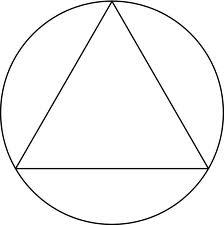Did you find an error or inaccuracy? Feel free to write us. Thank you!Tips to related online calculators

#### You need to know the following knowledge to solve this word math problem:

We encourage you to watch this tutorial video on this math problem:

## Related math problems and questions:

• n-gonWhat is the side length of the regular 5-gon inscribed in a circle of radius 12 cm?
• SAS triangleThe triangle has two sides long 7 and 19 and included angle 36°. Calculate area of this triangle.
• Chord - TSThe radius of circle k measures 68 cm. Arc GH = 47 cm. What is TS?
• TriangleCalculate the area of ​​the triangle ABC if b = c = 17 cm, R = 19 cm (R is the circumradius).
• Nine-gonCalculate the perimeter of a regular nonagon (9-gon) inscribed in a circle with a radius 13 cm.
• Hexagon ACalculate area of regular hexagon inscribed in circle with radius r=9 cm.
• Arc and segmentCalculate the length of circular arc l, area of the circular arc S1 and area of circular segment S2. Radius of the circle is 11 and corresponding angle is (2)/(12) π.
• Circular sector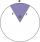I have a circular sector with a length 15 cm with an unknown central angle. It is inscribed by a circle with radius 5 cm. What is the central angle alpha in the circular sector?
• Equilateral triangle v3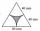Calculate the content of the colored gray part. Equilateral triangle has side length 8 cm. Arc centers are the vertices of a triangle.
• Regular n-gonWhich regular polygon have a radius of circumscribed circle r = 10 cm and the radius of inscribed circle p = 9.962 cm?
• n-gon IIWhat is the side length of the regular 5-gon circumscribed circle of radius 11 cm?
• RhombusIt is given a rhombus of side length a = 19 cm. Touchpoints of inscribed circle divided his sides into sections a1 = 5 cm and a2 = 14 cm. Calculate the radius r of the circle and the length of the diagonals of the rhombus.
• EQL triangle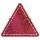Calculate the inradius and circumradius of an equilateral triangle with side a=77 cm.
• PentagonCalculate the length of side, circumference and area of a regular pentagon, which is inscribed in a circle with radius r = 6 cm.
• Block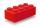Calculate the volume of a cuboid ABCDEFGH if |AB| = 16 cm, |BC| = 19 cm and the angle ∠CDG = 36.9°
• Hexagon 5The distance of parallel sides of regular hexagonal is 61 cm. Calculate the length of the radius of the circle described to this hexagon.
• Inscribed and described circle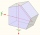Find the radii of a circle inscribed and circumscribed by a regular pentagon whose side measures 3 cm.